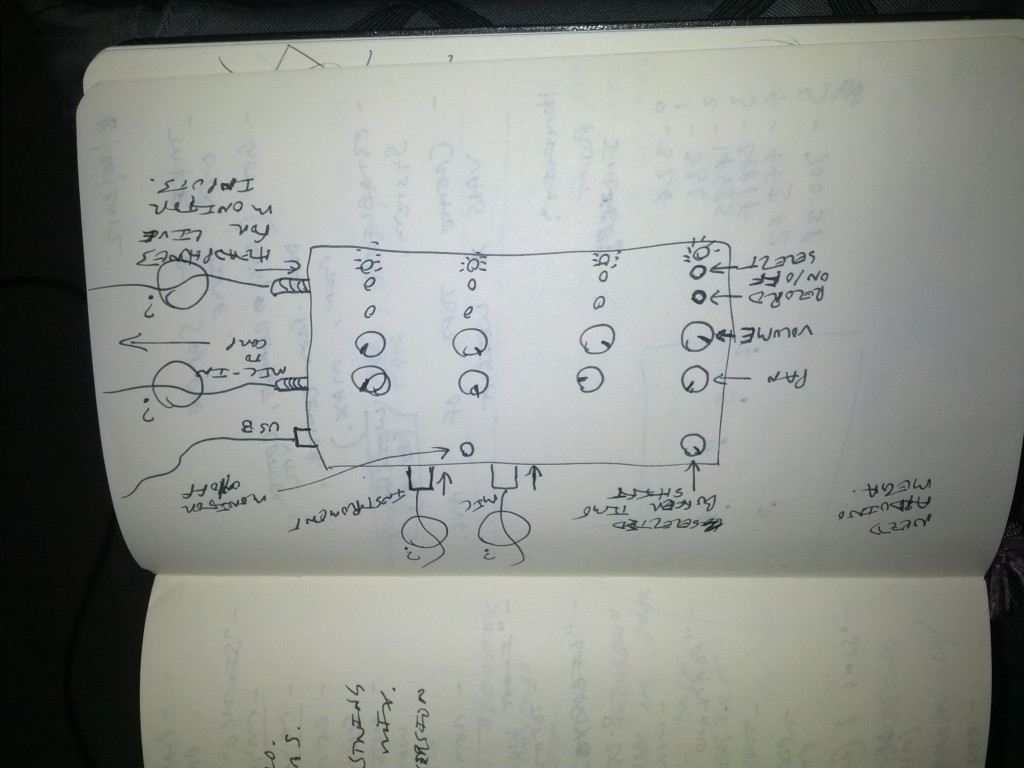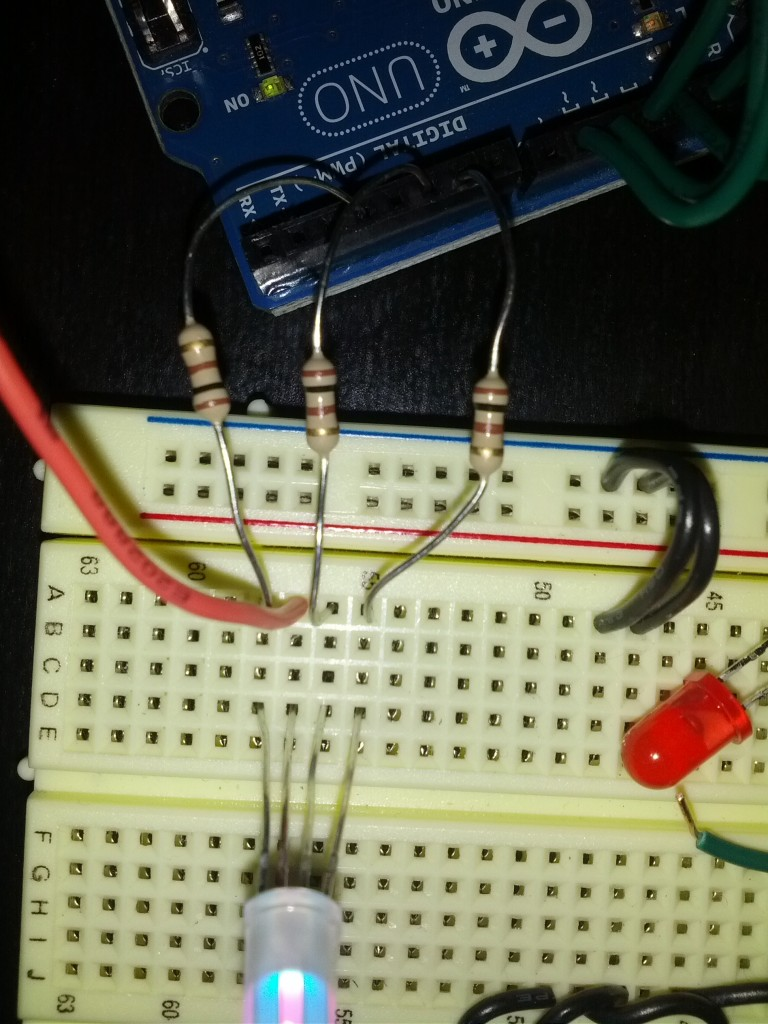# Music interface, Multi-colored LEDs, and Push button pots, OH MY!

First post in a while.

I am currently building an interface for controlling a MaxMSP patch for playing my music live. I want to be able to create loops in real-time from both instruments and ambient sounds, apply some processes to each channel (separate loops) and control the playback.

A sketch of my initial idea (this will change).I found some potentiometers that also have a push button on them, so I figured that the pot can be used to control a specific effect, and the button can be used to cycle through a selected effect to control. Therefore, I will have LEDs for each channel that will visually tell you which effect you have selected for that channel.

I have documented the LED learning process, not the POT yet, but I will get there.

I got this Full-color LED from radioshack > http://www.radioshack.com/product/index.jsp?productId=3020765. It is a common ANODE LED which means you must power the anode rather than grounding the common cathode. It was a bit tricky at first since it did not come with a wiring diagram. So, here is a picture of the wiring scheme that I am using (and it works!)And then, here is the Arduino sketch that I have written to test out the multi-color capabilities. The code is fairly commented and straight forward. If you have questions, drop a line in the comments

int pins[] = { 3,5,6 }; //R,G,B
int vals[] = { 255,255,255 }; //the values that will be written to each Pin
int nextVals[] = { 0,0,0 }; //the values that are stored in order to do color fading

long currMillis;
long previousMillis;
int interval = 250;

void setup(){

Serial.begin(9600);

for(int i=0;i<sizeof(pins);i++){
pinMode(pins[i], OUTPUT);
}
}

void loop(){

currMillis = millis();

if(currMillis – previousMillis > interval){
previousMillis = currMillis;
for(int i=0;i<3;i++){
//if “interval” has passed, create 3 new numbers for the LED to fade into
nextVals[i] = random(0,255);
}
}

smoothVals(nextVals);

for(int i=0;i<3;i++){
analogWrite(pins[i], vals[i]);
}
}
void smoothVals(int v[]){
//int newVals[] = currV;
for(int i=0;i<3;i++){
int diff = (int) abs(vals[i] – v[i]) / 6; //find out how far off we currently are from the “nextVals” values
if(vals[i] <= v[i]){
vals[i] = vals[i] + diff; //add the difference is we are lower
}
else if(vals[i] >= v[i]){
vals[i] = vals[i] – diff; //subtract the difference if we are higher
}
}
}